# Using Thermistors in Temperature Tracking Power Supplies

### Abstract

This article provides a simple, intuitive tutorial on negative temperature coefficient (NTC) thermistors and how to make basic use of them in general, and specifically in power supply regulators. A good example application is their use to cancel temperature effects on LCD display contrast. Two simple NTC thermistor linearizing techniques are shown, and regulator design procedures and examples demonstrate their application. Each example includes a schematic and compares the measured output voltage versus temperature to the target.

Power supply regulators, by definition, are designed to provide an output voltage that is stable despite variations in line (input voltage), load, and temperature. While for most applications, a stable output is the goal, there are some applications where it is advantageous to provide a temperature-dependent output voltage. This article provides a tutorial, design procedure, and circuit examples utilizing negative temperature coefficient (NTC) thermistors in temperature tracking power supplies.

By far the most common application for temperature-dependent regulation is in LCD bias supplies, where the contrast of the display will vary with ambient temperature. By applying a temperature-dependent bias voltage, the LCD's temperature effects can be automatically canceled to maintain constant contrast over a wide temperature range. The examples in this article are targeted toward LCD bias solutions; however, the tutorial and design equations are simple and may be easily applied in a variety of circuits.

### Why NTC Thermistor?

The NTC thermistor provides a near optimum solution for temperature-dependent regulation. It is low-cost, readily available through a variety of suppliers (Murata, Panasonic, etc.), and available in small surface-mount packaging from 0402 size through 1206 size. Furthermore, with only a basic understanding, the NTC thermistor is straightforward to apply to your circuit.

### NTC Characteristic

As the name implies, the thermistor is just a temperature-dependent resistor. Unfortunately, the dependence is very non-linear (see Figure 1) and, by itself, would not be very helpful for most applications. Fortunately, there are two easy techniques to linearize a thermistor's behavior.The standard formula for NTC thermistor resistance as a function of temperature is given by:where R25C is the thermistor's nominal resistance at room temperature, β (beta) is the thermistor's material constant in K, and T is the thermistor's actual temperature in Celsius.

This equation is a very close approximation of the actual temperature characteristic, as can be seen in Figure 2. Note the use of log-scale for the Y-axis.R25C and β are usually published in the manufacturer's data sheet. Typical values of R25C range from 22Ω to 500kΩ. Typical values of β are from 2500 to 5000K.

As seen in Figure 3, higher values of β provide increased temperature dependence and are useful when higher resolution is required over a narrower temperature range. Conversely, lower values of β offer less-sloped temperature dependence and are more desirable when operating over a wider temperature range.#### Self Heating

A thermistor is a resistor, and, just like any resistor, it produces heat energy whenever current passes through it. The heat energy causes the NTC thermistor's resistance to reduce which then indicates a temperature slightly above ambient temperature. In the manufacturer's data sheets and application notes, there are usually tables, formulae, and text detailing this phenomenon. However, these may be largely ignored if the current through the thermistor is kept relatively low such that self heating error is small compared to the required measurement accuracy, as in the design examples of this article.

### Linearizing

An NTC thermistor is most easily utilized when applied in a linearizing circuit. There are two simple techniques for linearization: resistance mode and voltage mode.

#### Resistance Mode

In resistance mode linearization, a normal resistor is placed in parallel with the NTC thermistor, which has the effect of linearizing the combined circuit's resistance. If the resistor's value is chosen to be equal to the thermistor's resistance at room temperature (R25C), then the region of relatively linear resistance will be symmetrical around room temperature (as seen in Figure 4).Note that lower values of β produce linear results over a wider temperature range, while higher values of β produce increased sensitivity over a narrower temperature range. The equivalent resistance varies from roughly 90% of R25C at cold (-20°C) to 50% of R25C at room temperature (+25°C) to roughly 15% of R25C at hot (+70°C).

#### Voltage Mode

In voltage mode linearization, the NTC thermistor is connected in series with a normal resistor to form a voltage-divider circuit. The divider circuit is biased with a regulated supply or a voltage reference, VREF. This has the effect of producing an output voltage that is linear over temperature. If the resistor's value is chosen to be equal to the thermistor's resistance at room temperature (R25C), then the region of linear voltage will be symmetrical around room temperature (as seen in Figure 5).Again, note that lower values of β produce linear results over a wider temperature range, while higher values of β produce increased sensitivity over a narrower temperature range. The output voltage varies from near zero volts at cold (-20°C) to VREF/2 at room (+25°C) to near VREF at hot (+70°C).

### Design Procedure

To create a regulated output voltage that varies linearly with temperature, the linearized thermistor circuit is applied to the regulator's feedback network.

#### Resistance Mode

The resistance mode circuit is the simplest solution for creating a temperature-dependent regulated output voltage because regulator feedback networks are almost always comprised of a resistive voltage divider. As seen in Figure 6, the linearized thermistor circuit is placed in series with one of the feedback resistors. In this case, the linearized circuit is placed in series with the top resistor of the feedback divider network to create a negative-temperature-coefficient output voltage at VOUT, as generally required in LCD bias solutions. (To create a positive-temperature-coefficient output, the linearizing circuit would be placed in series with the bottom resistor, R2, of the feedback divider.)The design procedure is relatively simple. First find the appropriate feedback network bias current, i2, from the regulator's data sheet. It is usually in the 10s to 100s of µA range and there is some latitude in its exact value. Then calculate the NTC thermistor value as:where TC is the negative temperature coefficient of VOUT in %/°C. The value of i2 should be adjusted until R25C becomes a readily available NTC thermistor value.

For a simplified design calculation, select R2 and R1 as: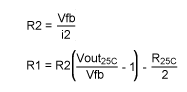where VFB is the nominal feedback voltage as given in the regulator's data sheet.

For a more accurate design calculation, the final value of i2 will end up being slightly modified in order to match the thermistor's β to the desired TC. Therefore, calculate the thermistor's resistance at 0°C and +50°C. The standard formula for NTC thermistor resistance as a function of temperature is given by:Then calculate the linearized resistance at the two temperatures as:Calculate the value of R2 and i2 as: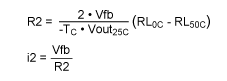And lastly calculate the value of R1 as:#### Resistance Mode Design Example

An LCD bias voltage is needed in a system running on a single-cell Li+ rechargeable battery. The desired bias voltage is VOUT = 20V at room temperature with T= -0.05%/°C. The MAX1605 regulator is selected for the task. The above design formulae are used to calculate the required components as follows:

Per the datasheet, i2 should be greater than 10uA for less than 1% output error; therefore, choose i2 to be about five times larger for less error:An NTC thermistor is chosen with R25C = 20kΩ and β = 3965K and linearized with a parallel 20kΩ resistor. The MAX1605 has a nominal feedback voltage of VFB = 1.25V. According to the simplified design formulae, R2 and R1 are then calculated as:Per the more accurate design calculation, the thermistor's resistance at 0°C and +50°C will be:The linearized resistances at 0°C and +50°C will be: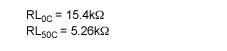The values for R2, i2, and R1 are then calculated as:In this case, these more accurate values are not substantially different from those obtained using the simplified calculations. The final circuit can be seen in Figure 7.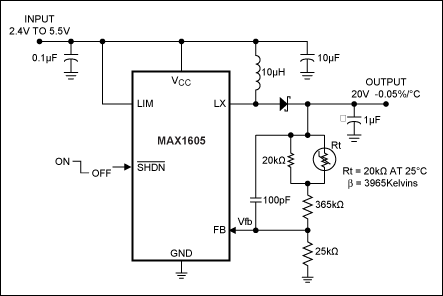The output voltage of the circuit of Figure 7 exhibits nearly ideal temperature dependence, as can be seen in Figure 8.#### Voltage Mode

Although more complicated than the resistance mode circuit, the voltage mode circuit has some unique advantages. First, the voltage mode circuit provides a temperature dependent analog voltage that may be easily digitized with an analog-to-digital converter (ADC) to provide temperature information to the system's microprocessor. Additionally, the regulator's output voltage temperature coefficient may be easily adjusted by changing the value of only one resistor. This benefit allows for simple trial-and-error design in the laboratory and may also be very valuable for accommodating multi-sourced thermistors or LCD panels in production.

As seen in Figure 9, the linearized thermistor circuit is biased with a voltage reference to generate a temperature-dependent voltage, VTEMP. Then, VTEMP is summed into the feedback node through a resistor, R3, which sets the gain of the temperature dependence. So that VTEMP does not need to be buffered, the nominal resistance of the thermistor should be kept much lower than R3. As connected in Figure 9, the regulator exhibits a negative-temperature-coefficient output voltage at VOUT, as generally required in LCD bias solutions. (To create a positive-temperature-coefficient output, the position of R and Rt should be reversed.)Although not mandatory, the simplest implementation of Figure 9 is when VREF = 2xVfb. (Conveniently, many regulators have VFB = 1.25V, many voltage references have VREF = 2.5V, and many ADCs have input voltage range from 0 to 2.5V.) When VREF = 2xVfb, VTEMP will equal VFB at +25°C and i3 will equal zero. This allows R1 and R2 to set the nominal output voltage at +25°C independent of R3 and the thermistor. Select R2 according to the recommendations in the regulator's data sheet. Then calculate R1 and i2 as:Then calculate the approximate value of R3 as:where TC is the negative temperature coefficient of VOUT in %/°C. (This value of R3 will suffice for a simplified design calculation and may be later adjusted through experimentation in the laboratory.) Then, to avoid the need for a buffer amplifier between VTEMP and R3, choose a nominal thermistor value of:For a more accurate calculation, the final value of R3 will end up being slightly modified in order to match the thermistor's β to the desired TC. To do this, first calculate the thermistor's resistance at 0°C and +50°C. The standard formula for NTC thermistor resistance as a function of temperature is given by:Then calculate the linearized voltage, VTEMP, at the two temperatures as:The more accurate value of R3 is finally given as:#### Voltage Mode Design Example

An LCD bias voltage is needed in a system running on a Li+ battery. The desired bias voltage is VOUT = 20V at room temperature with T= -0.05%/°C. The MAX629 regulator is selected for the task because it has a reference voltage output that may be used to bias the thermistor linearizing network. The voltage mode design formulae are used to calculate the required components as follows:

Per the datasheet, R2 should be in the range of 10kΩ to 200kΩ and VFB = 1.25V; therefore: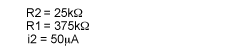The approximate value of R3 will be:The thermistor's nominal resistance should be kept less than 46.9kΩ. Therefore, an NTC thermistor is chosen with R25C = 20kΩ and β = 3965K and linearized with a series 20kΩ resistor and VREF = 2.5V bias.

Per the more accurate design calculation, the thermistor's resistance at 0°C and +50°C will be:The linearized voltage at 0°C and +50°C will be: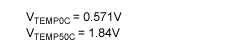The new value for R3 is then calculated to be: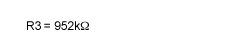In this case, the more accurate R3 value is not substantially different from the value obtained using the simplified calculations, and the nearest standard resistor value should be chosen.

#### Design Example when VREF ≠ 2xVfb

In the above voltage mode design example, if there isn't already a VREF = 2.5V supply in the system, it may be cost prohibitive to add one. Fortunately, any regulated voltage will suffice. For this example, the REF pin of the MAX629 is utilized and VREF' = 1.25V. Compared to the above example, VTEMP will now vary over half as wide a range; therefore, R3 must be halved to R3' = 475kΩ to maintain the same output voltage temperature coefficient of T= -0.05%/°C. Also, it is advisable to reduce the thermistor value and linearizing resistor value to R = R25C = 10kΩ. Furthermore, because VTEMP is lower than VFB at 25°C, i3 will be non-zero and the regulator's output voltage will be slightly higher than desired by: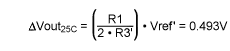To eliminate this, reduce R1 from 375kΩ to:The final circuit can be seen in Figure 10.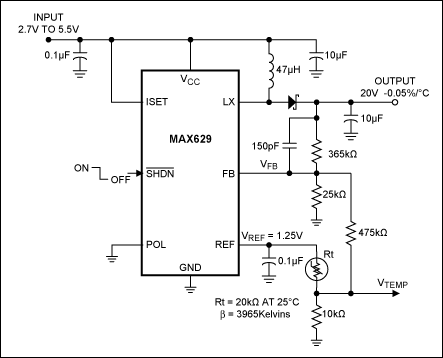The output voltage of the circuit of Figure 10 exhibits nearly ideal temperature dependence, as seen in Figure 11.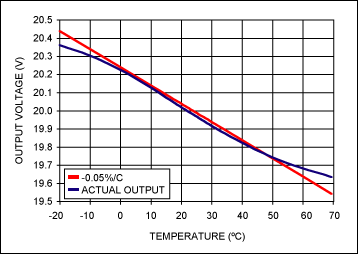A similar version of this article appeared in the August 1, 2001 issue of EDN magazine.